# Chapter TwentyEight Game Theory Game Theory u Game

• Slides: 45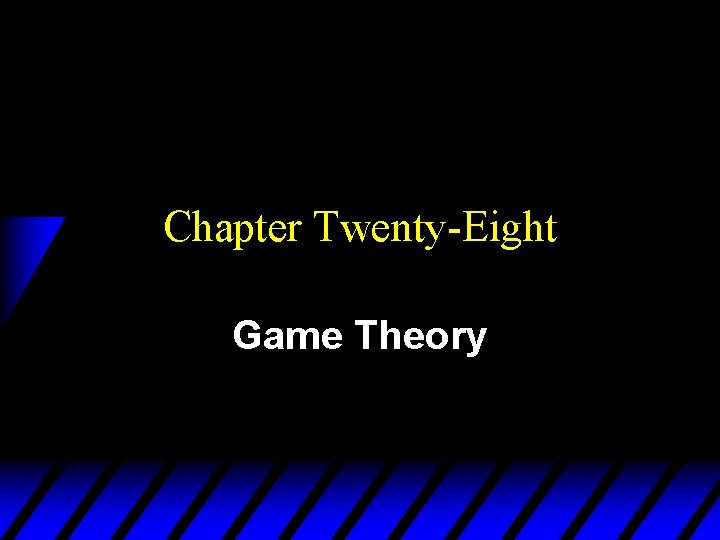Chapter Twenty-Eight Game Theory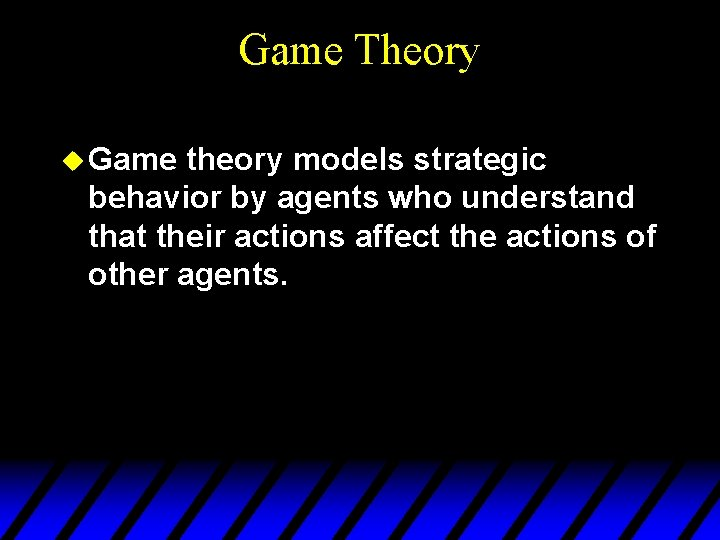Game Theory u Game theory models strategic behavior by agents who understand that their actions affect the actions of other agents.Some Applications of Game Theory u The study of oligopolies (industries containing only a few firms) u The study of cartels; e. g. OPEC u The study of externalities; e. g. using a common resource such as a fishery. u The study of military strategies.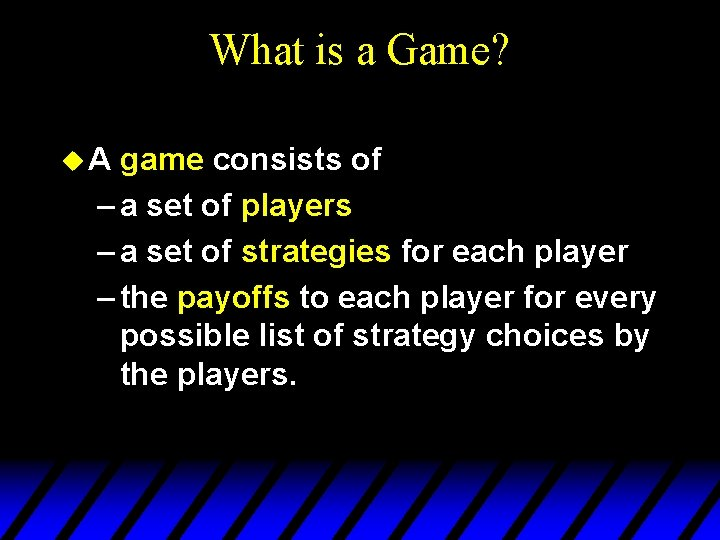What is a Game? u. A game consists of – a set of players – a set of strategies for each player – the payoffs to each player for every possible list of strategy choices by the players.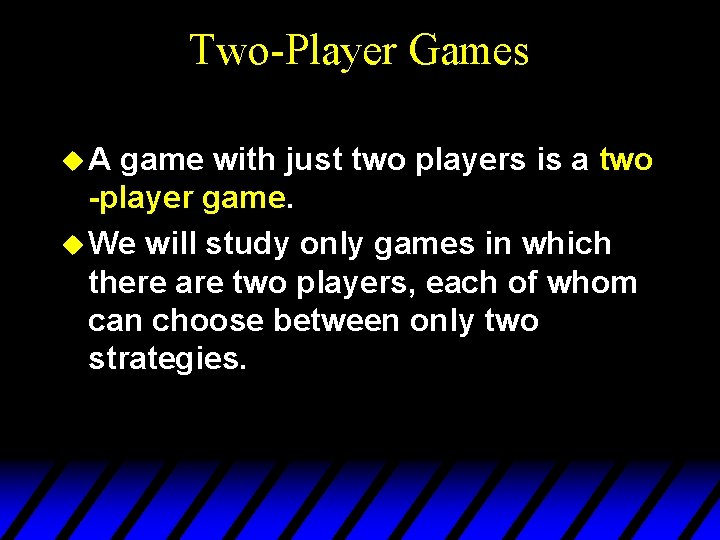Two-Player Games u. A game with just two players is a two -player game. u We will study only games in which there are two players, each of whom can choose between only two strategies.An Example of a Two-Player Game Player B L R Player A U (3, 9) (1, 8) D (0, 0) (2, 1) This is the game’s payoff matrix. Player A’s payoff is shown first. Player B’s payoff is shown second.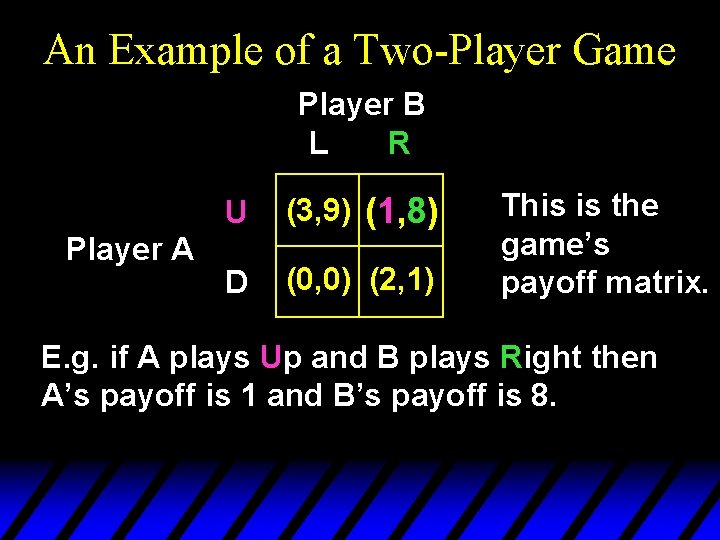An Example of a Two-Player Game Player B L R Player A U (3, 9) (1, 8) D (0, 0) (2, 1) This is the game’s payoff matrix. E. g. if A plays Up and B plays Right then A’s payoff is 1 and B’s payoff is 8.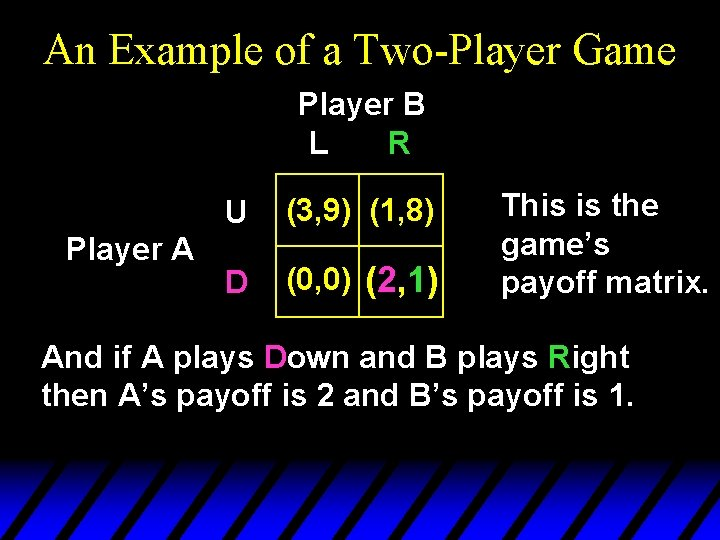An Example of a Two-Player Game Player B L R Player A U (3, 9) (1, 8) D (0, 0) (2, 1) This is the game’s payoff matrix. And if A plays Down and B plays Right then A’s payoff is 2 and B’s payoff is 1.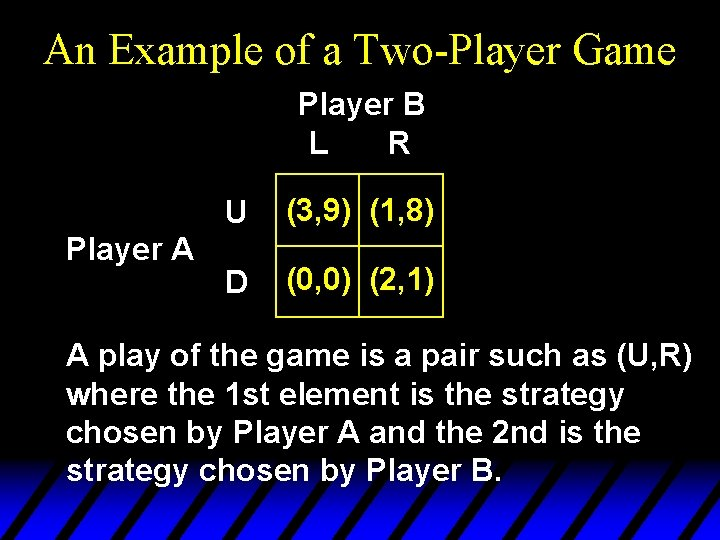An Example of a Two-Player Game Player B L R Player A U (3, 9) (1, 8) D (0, 0) (2, 1) A play of the game is a pair such as (U, R) where the 1 st element is the strategy chosen by Player A and the 2 nd is the strategy chosen by Player B.An Example of a Two-Player Game Player B L R Player A U (3, 9) (1, 8) D (0, 0) (2, 1) What plays are we likely to see for this game?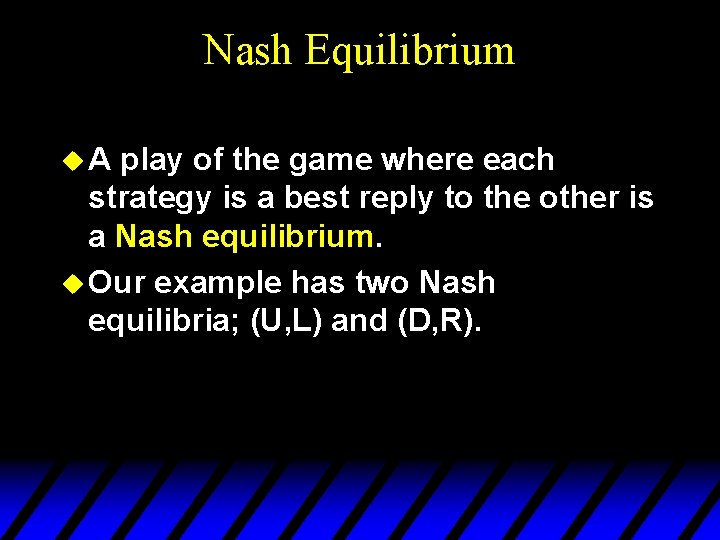Nash Equilibrium u. A play of the game where each strategy is a best reply to the other is a Nash equilibrium. u Our example has two Nash equilibria; (U, L) and (D, R).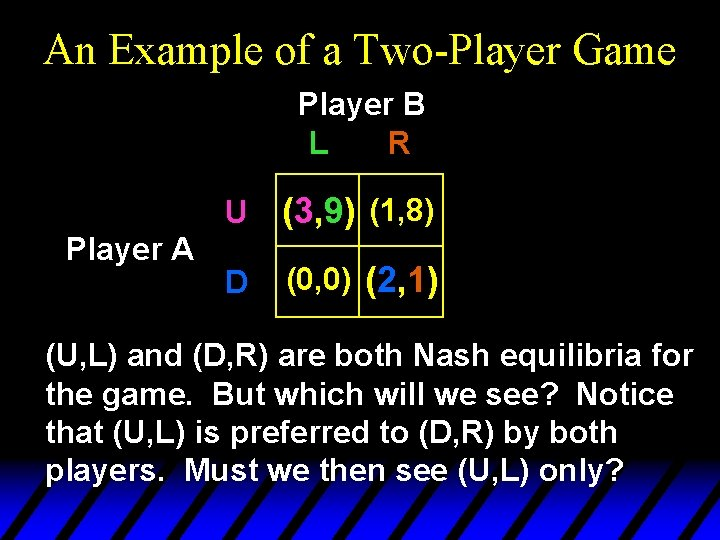An Example of a Two-Player Game Player B L R Player A U (3, 9) (1, 8) D (0, 0) (2, 1) (U, L) and (D, R) are both Nash equilibria for the game. But which will we see? Notice that (U, L) is preferred to (D, R) by both players. Must we then see (U, L) only?The Prisoner’s Dilemma u To see if Pareto-preferred outcomes must be what we see in the play of a game, consider a famous second example of a two-player game called the Prisoner’s Dilemma.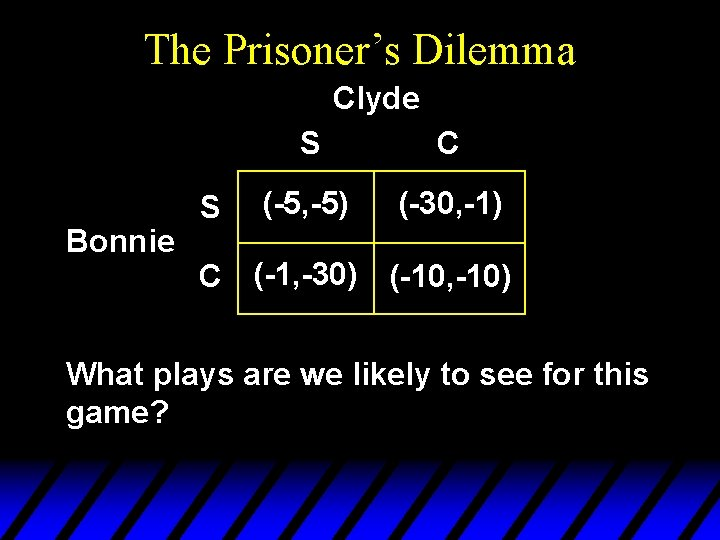The Prisoner’s Dilemma Clyde Bonnie S S C (-5, -5) (-30, -1) C (-1, -30) (-10, -10) What plays are we likely to see for this game?The Prisoner’s Dilemma Clyde Bonnie S S C (-5, -5) (-30, -1) C (-1, -30) (-10, -10) So the only Nash equilibrium for this game is (C, C), even though (S, S) gives both Bonnie and Clyde better payoffs. The only Nash equilibrium is inefficient.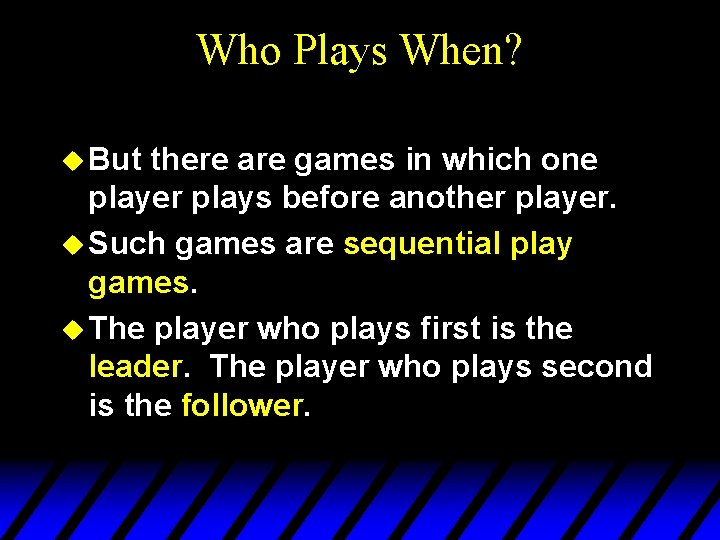Who Plays When? u But there are games in which one player plays before another player. u Such games are sequential play games. u The player who plays first is the leader. The player who plays second is the follower.A Sequential Game Example u Sometimes a game has more than one Nash equilibrium and it is hard to say which is more likely to occur. u When such a game is sequential it is sometimes possible to argue that one of the Nash equilibria is more likely to occur than the other.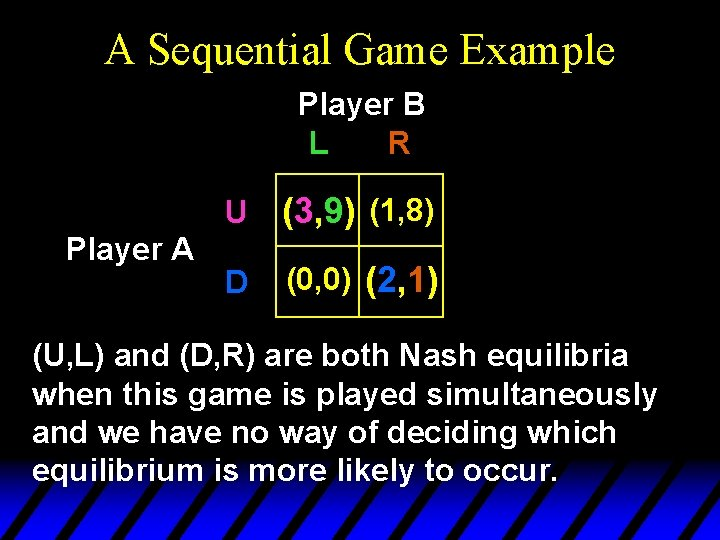A Sequential Game Example Player B L R Player A U (3, 9) (1, 8) D (0, 0) (2, 1) (U, L) and (D, R) are both Nash equilibria when this game is played simultaneously and we have no way of deciding which equilibrium is more likely to occur.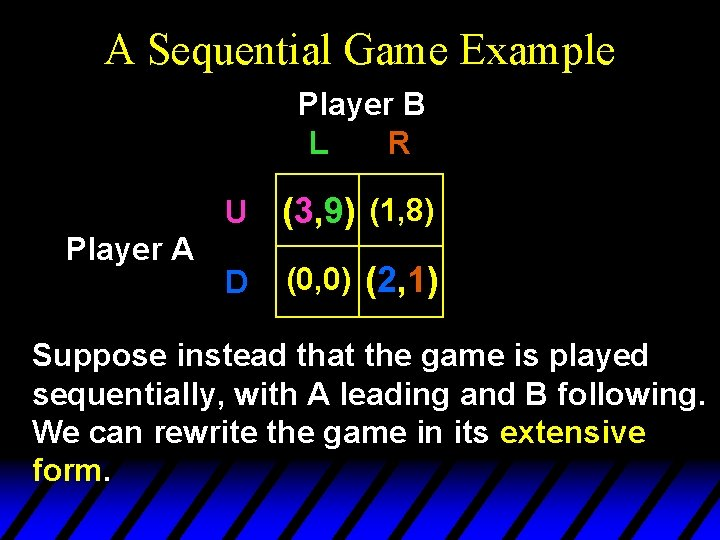A Sequential Game Example Player B L R Player A U (3, 9) (1, 8) D (0, 0) (2, 1) Suppose instead that the game is played sequentially, with A leading and B following. We can rewrite the game in its extensive form.A Sequential Game Example A U D B L (3, 9) A plays first. B plays second. B R L (1, 8) (0, 0) R (2, 1)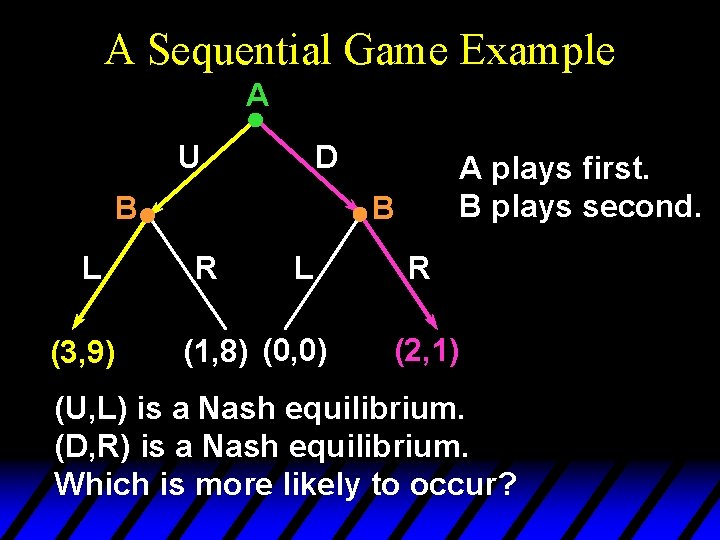A Sequential Game Example A U D B L (3, 9) A plays first. B plays second. B R L (1, 8) (0, 0) R (2, 1) (U, L) is a Nash equilibrium. (D, R) is a Nash equilibrium. Which is more likely to occur?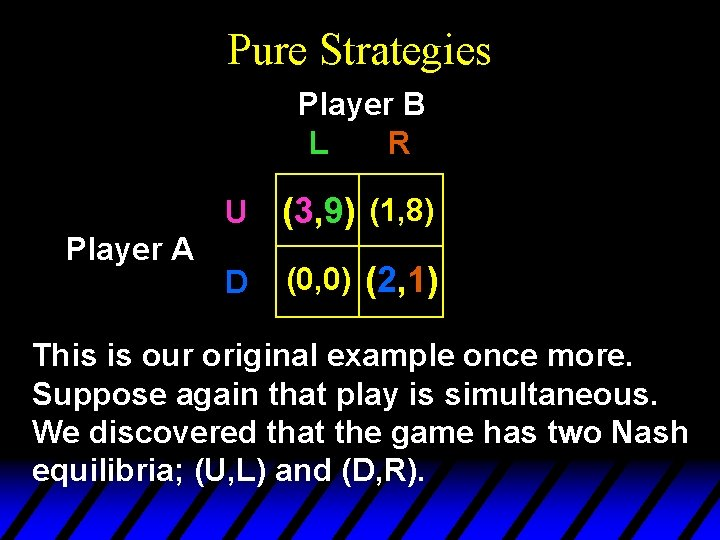Pure Strategies Player B L R Player A U (3, 9) (1, 8) D (0, 0) (2, 1) This is our original example once more. Suppose again that play is simultaneous. We discovered that the game has two Nash equilibria; (U, L) and (D, R).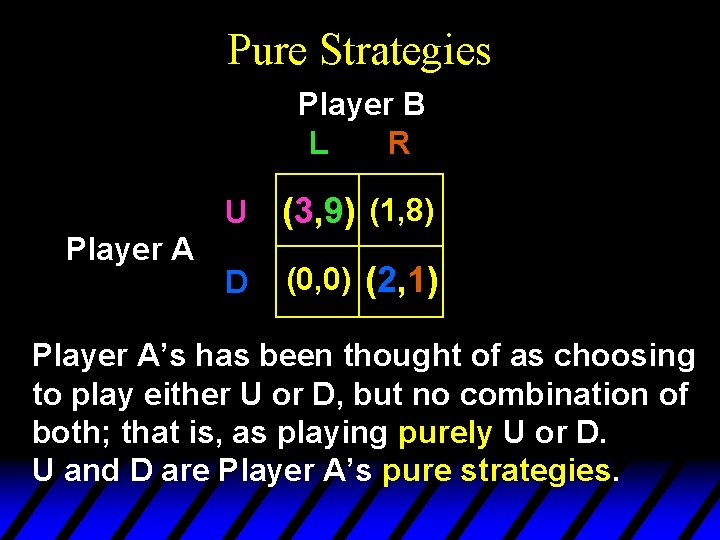Pure Strategies Player B L R Player A U (3, 9) (1, 8) D (0, 0) (2, 1) Player A’s has been thought of as choosing to play either U or D, but no combination of both; that is, as playing purely U or D. U and D are Player A’s pure strategies.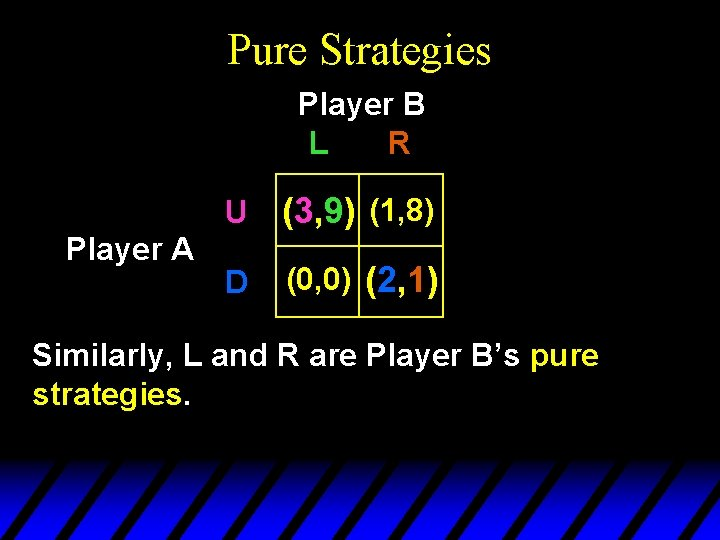Pure Strategies Player B L R Player A U (3, 9) (1, 8) D (0, 0) (2, 1) Similarly, L and R are Player B’s pure strategies.Pure Strategies Player B L R Player A U (3, 9) (1, 8) D (0, 0) (2, 1) Consequently, (U, L) and (D, R) are pure strategy Nash equilibria. Must every game have at least one pure strategy Nash equilibrium?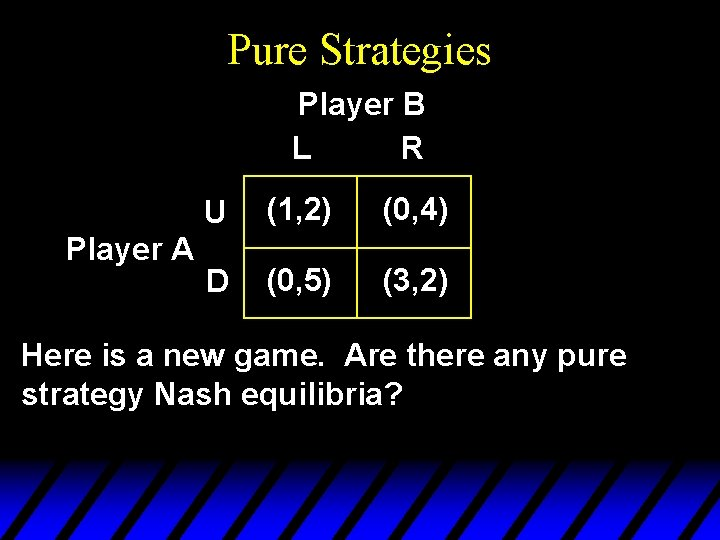Pure Strategies Player B L R Player A U (1, 2) (0, 4) D (0, 5) (3, 2) Here is a new game. Are there any pure strategy Nash equilibria?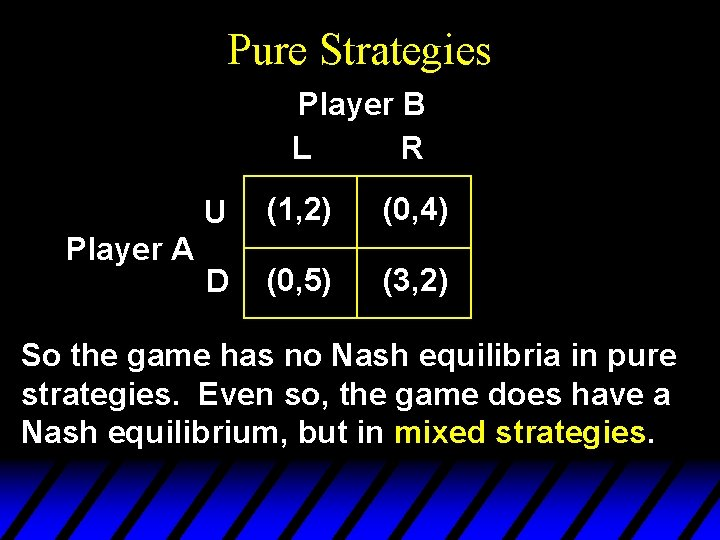Pure Strategies Player B L R Player A U (1, 2) (0, 4) D (0, 5) (3, 2) So the game has no Nash equilibria in pure strategies. Even so, the game does have a Nash equilibrium, but in mixed strategies.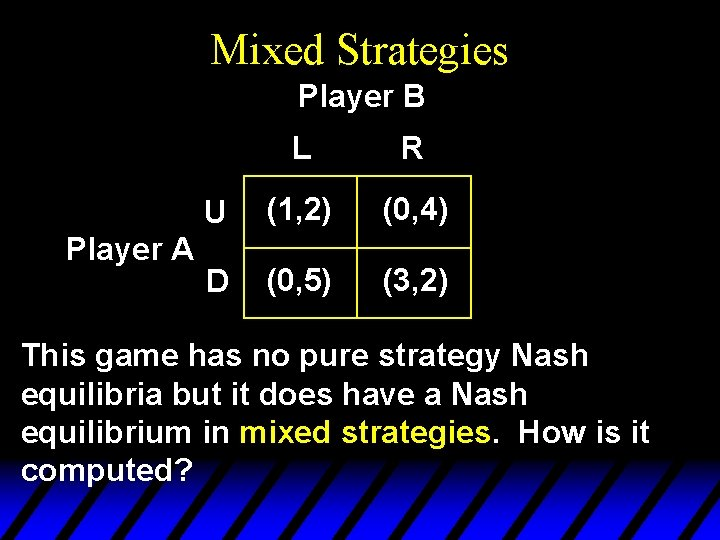Mixed Strategies Player B Player A L R U (1, 2) (0, 4) D (0, 5) (3, 2) This game has no pure strategy Nash equilibria but it does have a Nash equilibrium in mixed strategies. How is it computed?Mixed Strategies Player B U, p. U Player A D, 1 -p. U L, p. L R, 1 -p. L (1, 2) (0, 4) (0, 5) (3, 2)Mixed Strategies Player B U, p. U Player A D, 1 -p. U L, p. L R, 1 -p. L (1, 2) (0, 4) (0, 5) (3, 2) If B plays Left her expected payoff is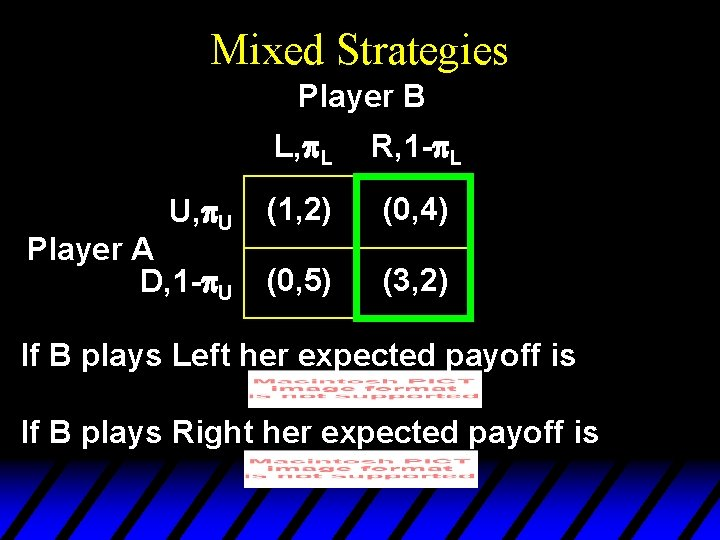Mixed Strategies Player B U, p. U Player A D, 1 -p. U L, p. L R, 1 -p. L (1, 2) (0, 4) (0, 5) (3, 2) If B plays Left her expected payoff is If B plays Right her expected payoff is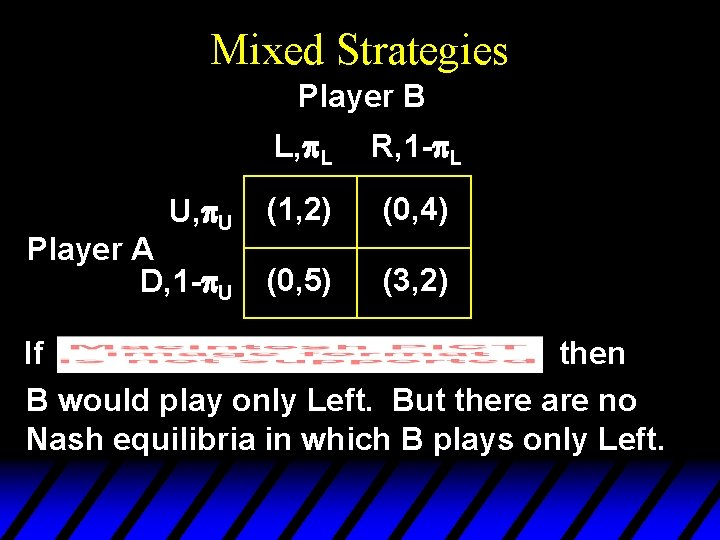Mixed Strategies Player B U, p. U Player A D, 1 -p. U L, p. L R, 1 -p. L (1, 2) (0, 4) (0, 5) (3, 2) If then B would play only Left. But there are no Nash equilibria in which B plays only Left.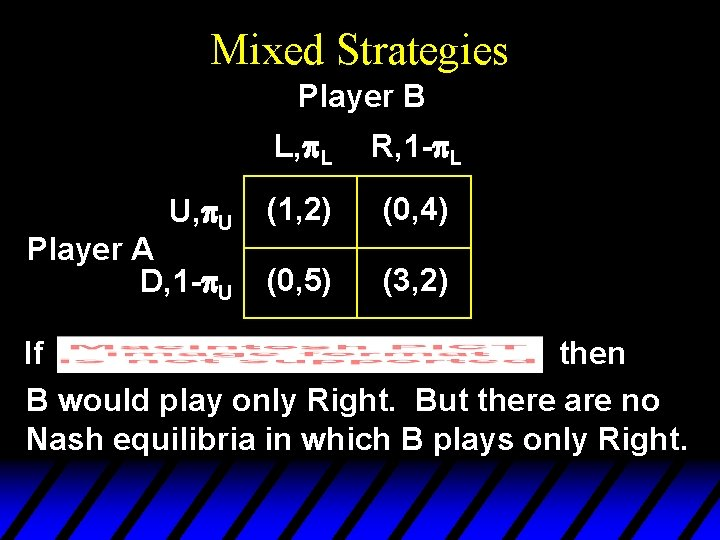Mixed Strategies Player B U, p. U Player A D, 1 -p. U L, p. L R, 1 -p. L (1, 2) (0, 4) (0, 5) (3, 2) If then B would play only Right. But there are no Nash equilibria in which B plays only Right.Mixed Strategies Player B U, p. U Player A D, 1 -p. U L, p. L R, 1 -p. L (1, 2) (0, 4) (0, 5) (3, 2) So for there to exist a Nash equilibrium, B must be indifferent between playing Left or Right; i. e.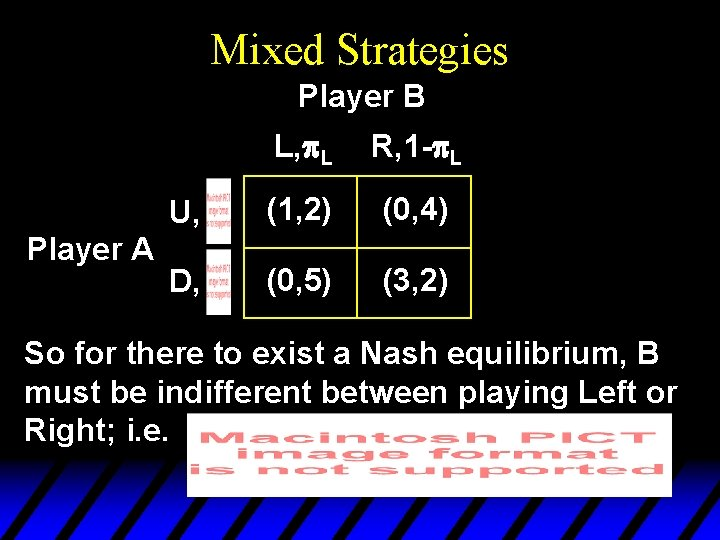Mixed Strategies Player B Player A L, p. L R, 1 -p. L U, (1, 2) (0, 4) D, (0, 5) (3, 2) So for there to exist a Nash equilibrium, B must be indifferent between playing Left or Right; i. e.Mixed Strategies Player B Player A L, R, U, (1, 2) (0, 4) D, (0, 5) (3, 2) So for there to exist a Nash equilibrium, A must be indifferent between playing Up or Down; i. e.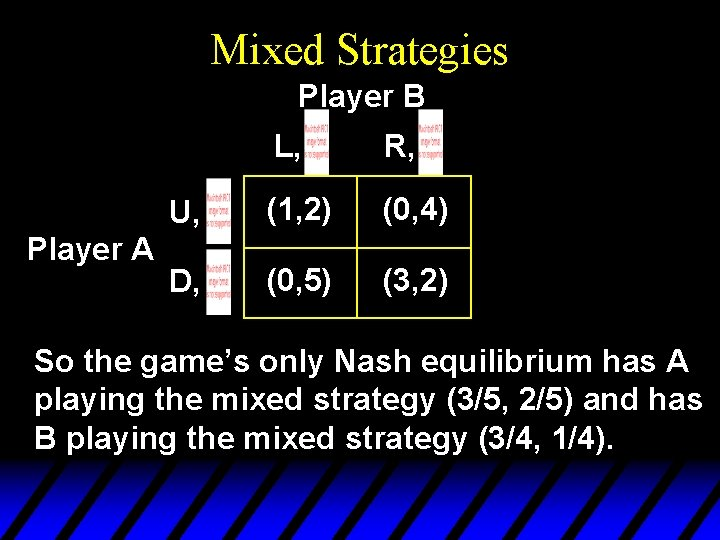Mixed Strategies Player B Player A L, R, U, (1, 2) (0, 4) D, (0, 5) (3, 2) So the game’s only Nash equilibrium has A playing the mixed strategy (3/5, 2/5) and has B playing the mixed strategy (3/4, 1/4).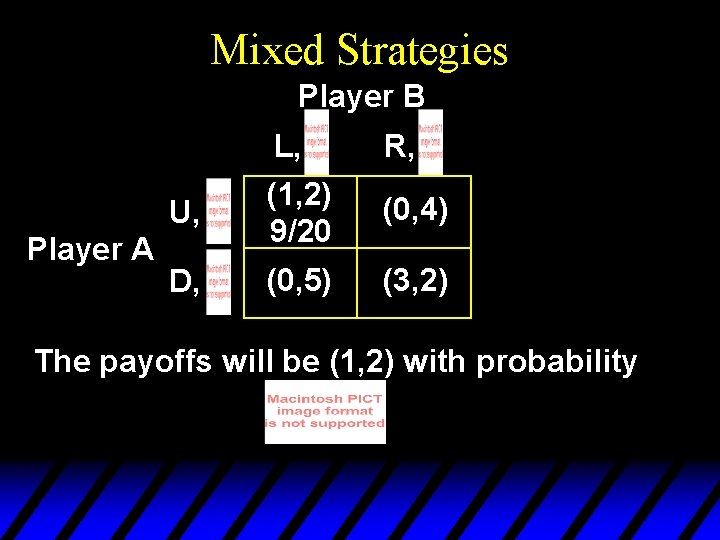Mixed Strategies Player B Player A L, R, U, (1, 2) 9/20 (0, 4) D, (0, 5) (3, 2) The payoffs will be (1, 2) with probabilityMixed Strategies Player B Player A L, R, U, (1, 2) 9/20 (0, 4) 3/20 D, (0, 5) (3, 2) The payoffs will be (0, 4) with probabilityMixed Strategies Player B U, Player A D, L, R, (1, 2) 9/20 (0, 5) 6/20 (0, 4) 3/20 (3, 2) The payoffs will be (0, 5) with probability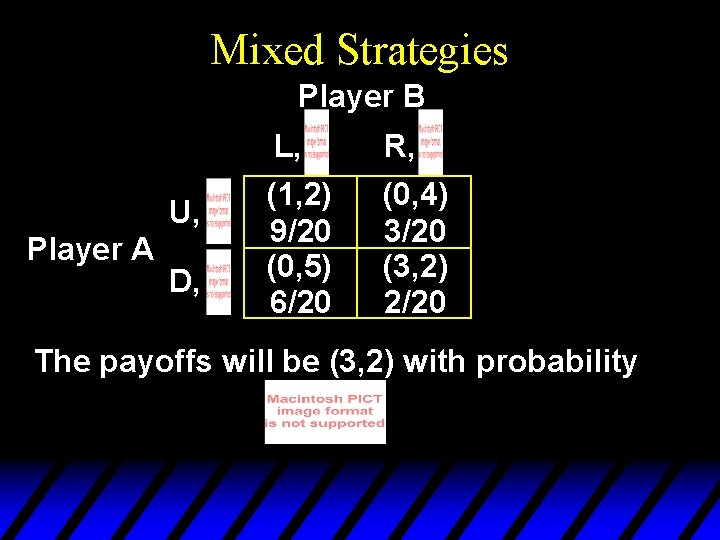Mixed Strategies Player B U, Player A D, L, R, (1, 2) 9/20 (0, 5) 6/20 (0, 4) 3/20 (3, 2) 2/20 The payoffs will be (3, 2) with probabilityMixed Strategies Player B U, Player A D, L, R, (1, 2) 9/20 (0, 5) 6/20 (0, 4) 3/20 (3, 2) 2/20Mixed Strategies Player B L, R, (1, 2) (0, 4) U, 9/20 3/20 Player A (0, 5) (3, 2) D, 6/20 2/20 A’s expected Nash equilibrium payoff is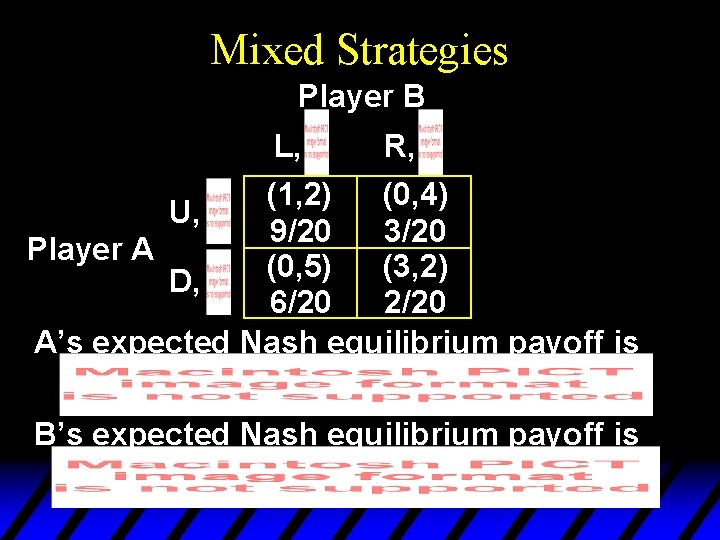Mixed Strategies Player B L, R, (1, 2) (0, 4) U, 9/20 3/20 Player A (0, 5) (3, 2) D, 6/20 2/20 A’s expected Nash equilibrium payoff is B’s expected Nash equilibrium payoff isHow Many Nash Equilibria? u. A game with a finite number of players, each with a finite number of pure strategies, has at least one Nash equilibrium. u So if the game has no pure strategy Nash equilibrium then it must have at least one mixed strategy Nash equilibrium.